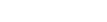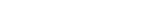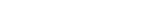Categories

# How to calculate the R-squared in Python with and without sklearnHow to calculate the R-squared in Python

This tutorial is about calculating the R-squared in Python with and without the sklearn package.

For an exemplary calculation we are first defining two arrays. While the y_hat is the predicted y variable out of a linear regression, the y_true are the true y values.

import numpy as np

y_hat = np.array([2,3,5,7,2,3,8,5,3,1])
y_true = np.array([5,4,2,7,4,2,1,6,5,3])



Now we are calculating the R-squared out of those two variables.

The formulas for calculating the R-squared are:where SST is:and SSE is:To understand the SST and SSE consider the following image found on Wikipedia and created by Orzetto (Please see the credits and license below the image):Attribution: Orzetto / CC BY-SA (https://creativecommons.org/licenses/by-sa/3.0)

On the left-hand side, you see the SST – the total sum of squares which are just the squared differences between the actual y values and the mean y.

On the right-hand side, you see the SSE – the residual sum of squares which is just the summed squared differences between the regression line (m*x+b) and the predicted y values.

You can also just use the sklearn package to calculate the R-squared.

from sklearn.metrics import r2_score

r2_score(y_true,y_hat)



For an application of the R-squared on real data, you are kindly invited to check out the video on my channel